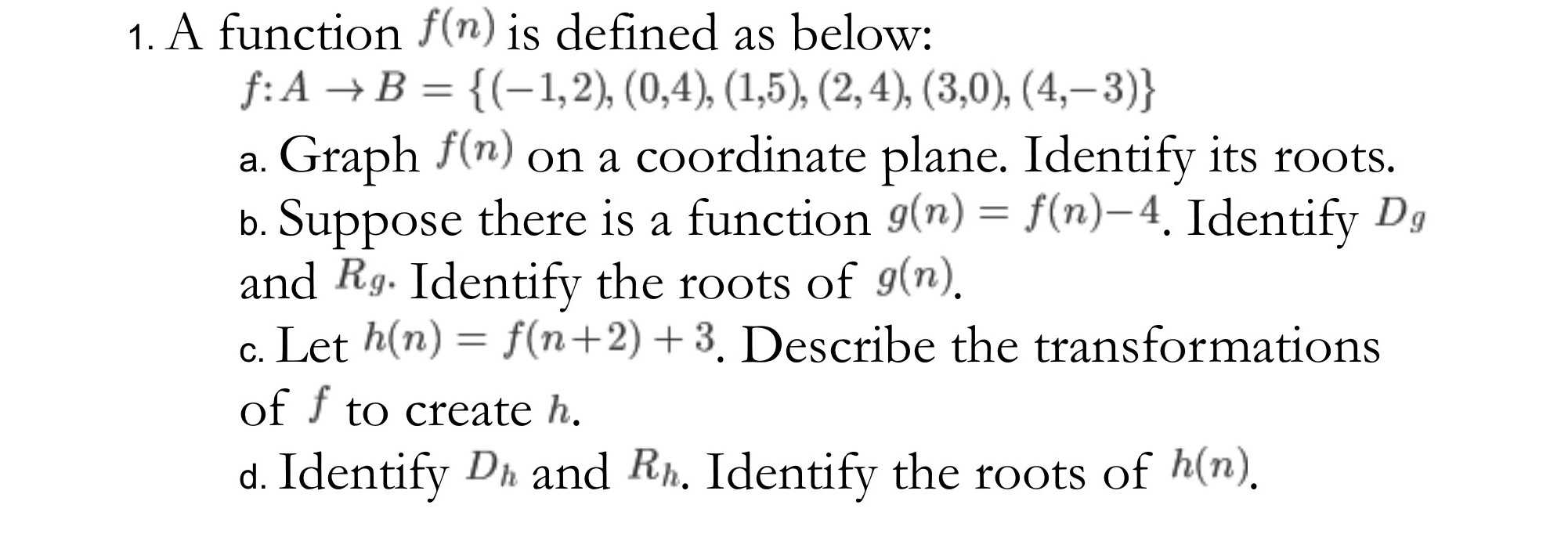### ¿Todavía tienes preguntas de matemáticas?

Pregunte a nuestros tutores expertos
Algebra
Pregunta1. A function $$f ( n )$$ is defined as below:

$$f : A \rightarrow B = \{ ( - 1,2 ) , ( 0,4 ) , ( 1,5 ) , ( 2,4 ) , ( 3,0 ) , ( 4 , - 3 ) \}$$

a. Graph $$f ( n )$$ on a coordinate plane. Identify its roots.

b. Suppose there is a function $$g ( n ) = f ( n ) - 4$$ . Identify $$D _ { g }$$ and $$R g .$$ Identify the roots of $$g ( n )$$ .

c. Let $$h ( n ) = f ( n + 2 ) + 3$$ . Describe the transformations of $$f$$ to create $$h$$ .

d. Identify $$D _ { h }$$ and $$R _ { h }$$ . Identify the roots of $$h ( n )$$